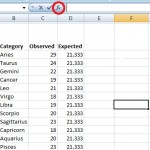# Chi Square P Value Excel: Easy Steps, Video

Share on

Watch the video on how to calculate a chi square p value in Excel.

P-values are used in hypothesis testing to help you figure out if your results are significant or not. A significant result is one where you reject the null hypothesis. In hypothesis testing, you’re really asking two questions:

• What do the results tell me about a population?
• What is the strength of those results?

A p-value is a number between 0 and 1, but it’s easier to think about them as percentages (i.e. a p-value of 0.05 is 5%). Small p-values (generally under 5%) usually lead you to reject the null hypothesis.

## Calculate the chi square p value Excel: Steps

Step 1: Calculate your expected value. The expected value in chi-square is found by dividing your counts (the number of responses or data items) by the number of categories. There are twelve categories (zodiac signs) in the question, so:
29 + 24 + 22 + 19 + 21 + 18 + 19 + 20 + 23 + 18 + 20 + 23 = 256
256 / 12= 21.333

Step 2: Type your data into columns in Excel. For this sample question, type your zodiac signs into column B, the observed values in column C (the observed values are the counts in the question) and your expected value (from Step 1) in column D.

Step 3: Click a blank cell anywhere on the worksheet and then click the “Insert Function” button on the toolbar.Step 4: Type “Chi” in the Search for a Function box and then click “Go.”

Step 5: Select “CHITEST” from the list and then click “OK.”

Step 6: Type a range into the “Actual Range” box for your observed values. For this sample problem, the observed values are in cells C3 to C14, so type “C3:C14.”

Step 7: Type a range into the “Expected Range” box for your expected values. For this sample problem, the observed values are in cells D3 to D14, so type “D3:D14.”

Step 8: Click “OK” to calculate the p-value in Excel, which for this example problem is .9265.

That’s how to find a chi square p value Excel!

## References

Glen, S. (2014). Excel for Statistics: Step by Step.

CITE THIS AS:
Stephanie Glen. "Chi Square P Value Excel: Easy Steps, Video" From StatisticsHowTo.com: Elementary Statistics for the rest of us! https://www.statisticshowto.com/probability-and-statistics/excel-statistics/calculate-chi-square-p-value-excel/
---------------------------------------------------------------------------Need help with a homework or test question? With Chegg Study, you can get step-by-step solutions to your questions from an expert in the field. Your first 30 minutes with a Chegg tutor is free!